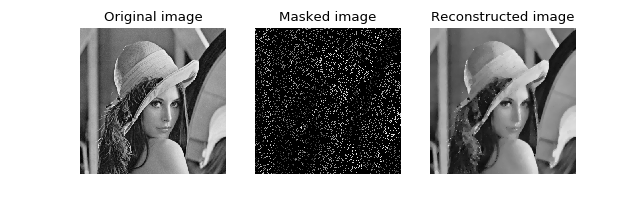# Image reconstruction (Forward-Backward, Total Variation, L2-norm)¶

This tutorial presents an image reconstruction problem solved by the Forward-Backward splitting algorithm. The convex optimization problem is the sum of a data fidelity term and a regularization term which expresses a prior on the smoothness of the solution, given by

$\min\limits_x \tau \|g(x)-y\|_2^2 + \|x\|_\text{TV}$

where $$\|\cdot\|_\text{TV}$$ denotes the total variation, y are the measurements, g is a masking operator and $$\tau$$ expresses the trade-off between the two terms.

Load an image and convert it to grayscale

>>> import matplotlib.image as mpimg
>>> import numpy as np
>>> try:
... except:
>>> im_original = np.dot(im_original[..., :3], [0.299, 0.587, 0.144])


and generate a random masking matrix

>>> np.random.seed(14)  # Reproducible results.


which masks 85% of the pixels. The masked image is given by

>>> g = lambda x: mask * x


The prior objective to minimize is defined by

$f_1(x) = \|x\|_\text{TV}$

which can be expressed by the toolbox TV-norm function object, instantiated with

>>> from pyunlocbox import functions
>>> f1 = functions.norm_tv(maxit=50, dim=2)


The fidelity objective to minimize is defined by

$f_2(x) = \tau \|g(x)-y\|_2^2$

which can be expressed by the toolbox L2-norm function object, instantiated with

>>> tau = 100
>>> f2 = functions.norm_l2(y=im_masked, A=g, lambda_=tau)


Note

We set $$\tau$$ to a large value as we trust our measurements and want the solution to be close to them. For noisy measurements a lower value should be considered.

The step size for optimal convergence is $$\frac{1}{\beta}$$ where $$\beta=2\tau$$ is the Lipschitz constant of the gradient of $$f_2$$ [BT09a]. The Forward-Backward splitting algorithm is instantiated with

>>> from pyunlocbox import solvers
>>> solver = solvers.forward_backward(step=0.5/tau)


and the problem solved with

>>> x0 = np.array(im_masked)  # Make a copy to preserve im_masked.
>>> ret = solvers.solve([f1, f2], x0, solver, maxit=100)
Solution found after 93 iterations:
objective function f(sol) = 4.268861e+03
stopping criterion: RTOL


Let’s display the results:

>>> import matplotlib.pyplot as plt
>>> fig = plt.figure(figsize=(8, 2.5))
>>> ax1 = fig.add_subplot(1, 3, 1)
>>> _ = ax1.imshow(im_original, cmap='gray')
>>> _ = ax1.axis('off')
>>> _ = ax1.set_title('Original image')
>>> ax2 = fig.add_subplot(1, 3, 2)